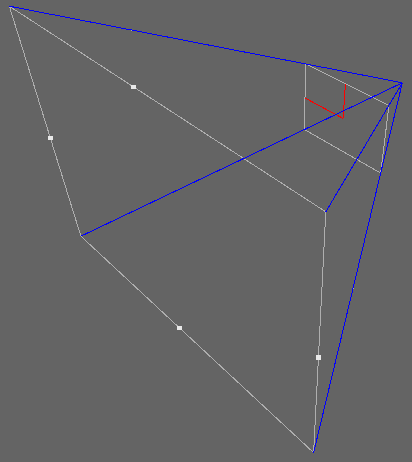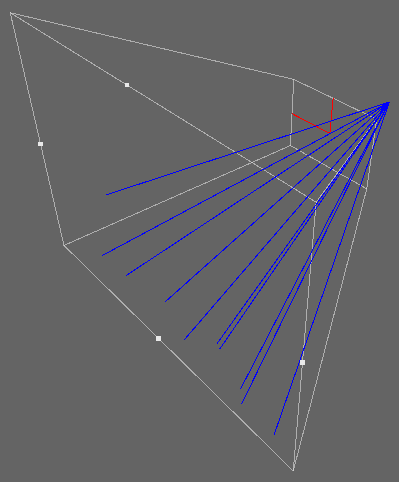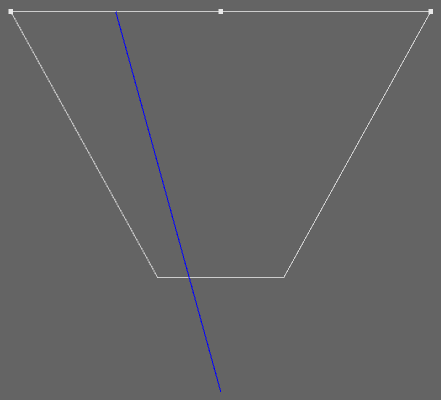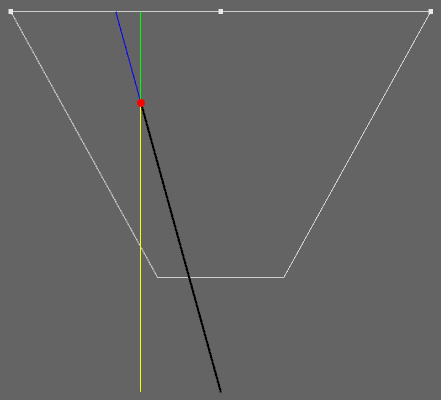# Jim's GameDev Blog# 通过深度图重建坐标

2016-12-6

### 重建相机空间坐标

$\begin{bmatrix} {cot{\theta \over 2} \over Aspect} & 0 & 0 & 0 \\ 0 & cot{\theta \over 2} & 0 & 0 \\ 0 & 0 & -{f+n \over f-n} & -{2nf \over f-n} \\ 0 & 0 & -1 & 0 \end{bmatrix}$

Matrix4x4 projectionMatrix = camera.projectionMatrix;
Vector3 posInCamSpace = myPos;
Vector3 posInNDC = projectionMatrix.MultiplyPoint(posInCamSpace);


MultiplyPoint 这个方法是引擎封装好了的，具体的实现是这样的：

public Vector3 MultiplyPoint(Matrix4x4 mat, Vector3 v)
{
Vector3 result;
result.x = mat.m00 * v.x + mat.m01 * v.y + mat.m02 * v.z + mat.m03;
result.y = mat.m10 * v.x + mat.m11 * v.y + mat.m12 * v.z + mat.m13;
result.z = mat.m20 * v.x + mat.m21 * v.y + mat.m22 * v.z + mat.m23;
float num = mat.m30 * v.x + mat.m31 * v.y + mat.m32 * v.z + mat.m33;
num = 1 / num;
result.x *= num;
result.y *= num;
result.z *= num;
return result;
}


public Vector3 MultiplyPoint(Matrix4x4 mat, Vector3 v)
{
Vector3 result;
result.x = mat.m00 * v.x;
result.y = mat.m11 * v.y;
float num = mat.m32 * v.z;
num = 1 / num;
result.x *= num;
result.y *= num;
return result;
}


posInCamSpace.x = posInNDC.x * zLinearEyeDepth / ProjMat;
posInCamSpace.y = posInNDC.y * zLinearEyeDepth / ProjMat;


float2 proj0011 = float2(unity_CameraProjection, unity_CameraProjection);
posInCamSpace.xy = posInNDC.xy * zLinearEyeDepth / proj0011;


posInCamSpace.xy = poseInNDC.xy * _OneOverProj0011 * zLinearEyeDepth;


### 重建世界空间坐标${YellowL \over YellowL + GreenL} = {\overrightarrow{BlackV} \over \overrightarrow{BlackV} + \overrightarrow{BlueV}}$

$YellowL + GreenL = 1$

$\overrightarrow{BlackV} = YellowL * \left( \overrightarrow{BlackV} + \overrightarrow{BlueV} \right)$

\begin{align} \overrightarrow{BlackV} &= YellowL * \overrightarrow{interpolatedRay} \\ wPos &= camWPos + \overrightarrow{BlackV} \end{align}